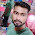### Program 170:To Print value of Sine Mathematically and using Library Function

Program 170:

```#include <stdio.h>
#include <math.h>
int factorial(int);
main()
{
//sinx=x1/1!-x3/3!+x5/5!-x7/7!...
int accuracy,count=0,k=1;
float x,temp,sine=0;

printf("Enter value x(in degrees) to find sin x value\n");
scanf("%f",&x);
temp=x;
x=(float)x*(3.14159/180);
printf("Enter value to get more accurate\n 3 for least and more for more accuracy\n");
scanf("%d",&accuracy);

do
{
if(count%2==0)
{
sine=(float)sine+(pow(x,k)/factorial(k));
}
if(count%2==1)
{
sine=(float)sine-(pow(x,k)/factorial(k));
}
count++;
k=k+2;
}while(count<=accuracy);

printf("Sine(%f)=%f calcualted mathematically\n",temp,sine);
printf("Sine(%f)=%f calculated using library function\n",temp,sin(x));
}

int factorial(int val)
{
int factorial=1,i;
for(i=1;i<=val;i++)
{
factorial=factorial*i;
}
return(factorial);

}
```
Explanation:

To know the sine value of any thing say 'x'.
Formula is
`sinx=x1/1!-x3/3!+x5/5!-x7/7!...`
1. The Program Starts with intializing
• accuracy→ To print output accurately
• count → To keep track of even and odd iterations
• k → To store power of a base
• x→ To store user input for substituting in place of x in formula
• temp temporary variable
• sine → To store output
2. ```printf("Enter value x(in degrees) to find sin x value\n");
scanf("%f",&x);
temp=x;```
Taking user input in 'x' and store it in temp for future reference as x may be manipulated while execution of program which cause the loss of original value in x
3. `x=(float)x*(3.14159/180); `
Value which we give in x is in terms of degrees so we need a conversion to radians.So value in x is converted to radians.Lets take value of x as 90(degrees) so x is converted to 1.570795 radians
4.
```do
{
if(count%2==0)
{
sine=(float)sine+(pow(x,k)/factorial(k));
}
if(count%2==1)
{
sine=(float)sine-(pow(x,k)/factorial(k));
}
count++;
k=k+2;
}while(count<=accuracy);```
In this logic we will calculate factorial of a number by passing the value(say k) to factorial(int val) function.The factorial function is:-
5. ```int factorial(int val)
{
int factorial=1,i;
for(i=1;i<=val;i++)
{
factorial=factorial*i;
}
return(factorial);

}```
This function takes the value(say k) from main and returns the factorial of received number to main.To clearly understand how this factorial function works click here to go to factorial program
6. Lets continue with our logic
7. ```do
{
if(count%2==0)
{
sine=(float)sine+(pow(x,k)/factorial(k));
}
if(count%2==1)
{
sine=(float)sine-(pow(x,k)/factorial(k));
}
count++;
k=k+2;
}while(count<=accuracy);
```
Lets take x as 90 degrees which is converted to 1.570795 radians .So to calculate sin(90).Here we used do while which executes atleast 1 time.
• Iteration 1:count and sine are initialized to zero.k initialized to 1.Lets take accuracy=3
```if(count%2==0)
{
sine=(float)sine+(pow(x,k)/factorial(k));
}```
• As count=0 so 0%2 is 0 which is true so if part executes
• And the value of sine=0+pow(1.570795,1)/1!→ sine=1.570795.
• Now the second if part is checked and as 0%2 !=1 so the part won't execute
``` if(count%2==1)
{
sine=(float)sine-(pow(x,k)/factorial(k));
}```
• Then both count is incremented by 1 and k is incremented by 2.
• So the final values after 1st iteration is x=1.570795,sine=1.570795,count=1;k=3
• Then count<=accuracy → 1<=3 which is true so the loop executes once more
• Iteration 2:
```if(count%2==0)
{
sine=(float)sine+(pow(x,k)/factorial(k));
}```
• As count=1 after 1st iteration so 1%2 != 0 so 1st if part won't executes
• Now the second if part is checked and as 1%2 =1 so the 2nd if part is executed
``` if(count%2==1)
{
sine=(float)sine-(pow(x,k)/factorial(k));
} ```
• And the value of sine=1.570795-pow(1.570795,3)/3!→ sine=0.915965158
• Then both count is incremented by 1 and k is incremented by 2.
• So the final values after 1st iteration is x=1.570795,sine=0.915965158,count=2;k=5
• Then count<=accuracy → 2<=3 which is true so the loop executes once more
• Iteration 3:
```if(count%2==0)
{
sine=(float)sine+(pow(x,k)/factorial(k));
}```
• As count=2 so 2%2 is 0 which is true so if part executes
• And the value of sine=0.915965158+pow(1.570795,5)/5!→ sine=0.995657448
• Now the second if part is checked and as 0%2 !=1 so the part won't execute
``` if(count%2==1)
{
sine=(float)sine-(pow(x,k)/factorial(k));
}```
• Then both count is incremented by 1 and k is incremented by 2.
• So the final values after 1st iteration is x=1.570795,sine=0.995657448,count=3;k=7
• Then count<=accuracy → 3<=3 which is true so the loop executes once more
• Iteration 4:
```if(count%2==0)
{
sine=(float)sine+(pow(x,k)/factorial(k));
}```
• As count=3 after 1st iteration so 3%2 != 0 so 1st if part won't executes
• Now the second if part is checked and as 3%2 =1 so the 2nd if part is executed
``` if(count%2==1)
{
sine=(float)sine-(pow(x,k)/factorial(k));
} ```
• And the value of sine=0.995657448-pow(1.570795,7)/7!→ sine=0.990975722~1.00(this is human calc so might not be as exact as system calc).
• Then both count is incremented by 1 and k is incremented by 2.
• So the final values after 1st iteration is x=1.570795,sine=1.00,count=4;k=9
• Then count<=accuracy → 4<=3 which is false so the loop is terminated
• Note:-
```In the given output I took 6 and 4 as accuracy but here we took it as 3 .
So more number of iterations we get more accuarate answer.
If we give accuracy as 6 .
The loop will iterate 3 more times same as above iterations.```
• So the final value of sine is printed

Output:

After 1st Run:

After 2nd Run:

#### 1 comment:

1.college-party-guide One of the most important rituals for students in college is to throw the most memorable student dorm celebration.
factorial hundred In the last few days, the “factorial of 100” is one of the top subjects and a lot of maths geeks compute it using voice assistants such as Alexa, Shiri, etc.
factorial hundred In the last few days, the “factorial of 100” is one of the top subjects and a lot of maths geeks compute it using voice assistants such as Alexa, Shiri, etc.
gigolo The term”gigolo” usually refers to the person who lives an arranged lifestyle that includes many of these relationships in a series, rather than having any other support system.